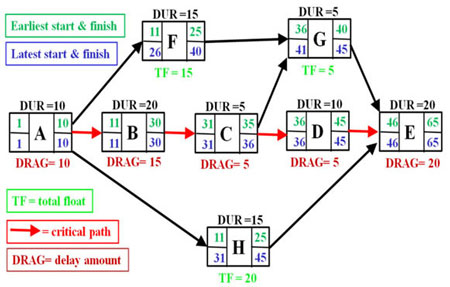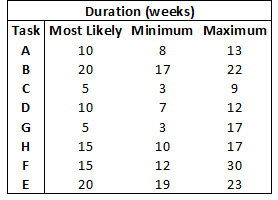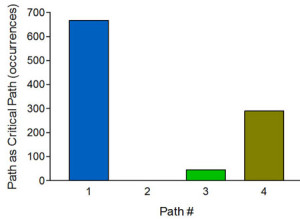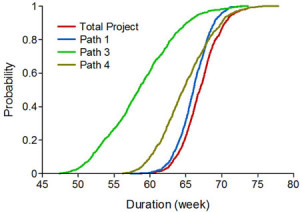# Project Management under Uncertainty

You can’t manage what you can’t measure
You can’t measure what you can’t define
How do you define something that isn’t known?

DeMarco, 1982

## 1.     Introduction

By the term Project we usually understand a unique, one-time operation designed to accomplish a set of objectives in a limited time frame. This could be building a new production plant, designing a new product or develop new software for a specific purpose.

A project usually differ from normal operations by; being a onetime operation, having a limited time horizon and budget, having unique specifications and by working across organizational boundaries. A project can be divides into four phases: project definition, planning, implementation and project phase-out.

## 2.     Project Scheduling

The project planning phase, which we will touch upon in this paper, consists of braking down the project into tasks that must be accomplished for the project to be finished.

The objectives of the project scheduling are to determine the earliest start and finish of each task in the project. The aim is to be able to complete the project as early as possible and to calculate the likelihood that the project will be completed within a certain time frame.

The dependencies[i] between the tasks determine their predecessor(s) and successor(s) and thus their sequence (order of execution) in the project. The aim is to list all tasks (project activities), their sequence and duration (estimated activity time length). The figure[ii] below shows a simple project network diagram, and we will in the following use this as an example[iii].This project thus consists of a linear flow of coordinated tasks where in fact time, cost and performance can vary randomly.

A convenient way of organizing this information is by using a Gantt[iv] chart. This gives a graphic representation of the project’s tasks, the expected time it takes to complete them, and the sequence in which they must be done.

There will usually be more than one path (sequence of tasks) from the first to the last task in a project. The path that takes the longest time to complete is the projects critical path. The objective of all this is to identify this path and the time it takes to complete it.

## 3.     Critical Path Analysis

The Critical Path (CP)[v] is defined as the sequence of tasks that, if delayed – regardless of whether the other project tasks are completed on or before time – would delay the entire project.

The critical path is hence based on the forecasted duration of each task in the project. These durations are given as single point estimates implying that the project’s tasks duration contain no uncertainty (deterministic). This is obviously wrong and will often lead to unrealistic project estimates due to the inherent uncertainty in all project work.

Have in mind that: All plans are estimates and are only as good as the task estimates.

As a matter of fact many different types of uncertainty can be expected in most projects:

1. Ordinary uncertainty, where time, cost and performance can vary randomly, but inside predictable ranges. Variations in task durations will cause the projects critical path to shift, but this can be predicted and the variation in total project time can be calculated.
2. Foreseen uncertainty, where a few known factors (events) can affect the project but in an unpredictable way. This is projects where tasks and events occur probabilistic and contain logical relationships of a more complicated nature. E.g. from a specific event some tasks are undertaken with certainty while others probabilistically (Elmaghraby, 1964) and (Pritsker, 1966). The distribution for total project time can still be calculated, but will include variation from the chance events.
3. Unforeseen uncertainty, where one or more factors (events) cannot be predicted. This will imply that decisions points about the projects implementation have to be included at one or more points in the projects execution.

As a remedy to the critical path analysis inadequacy to the existence of ordinary uncertainty, the Program Evaluation and Review Technique (PERT[vi]) analysis was developed. PERT is a variation on Critical Path Analysis that takes a slightly more skeptical view of the duration estimates made for each of the project tasks.

PERT uses a tree-point estimate,[vii] based on the forecast of the shortest possible task duration, the most likely task duration and the worst-case task duration. The tasks expected duration is then calculated as a weighted average of these tree estimates of the durations.

This is assumed to help to bias time estimates away from the unrealistically short time-scales that often is the case.

## 4.     CP, PERT and Monte Carlo Simulation

The two most important questions we want answered are:

• How long will it take to do the project?
• How likely is the project to succeed within the allotted time frame?
• In this example the projects time frame is set to 67 weeks.

We will use the Critical Path method, PERT and Monte Carlo simulation to try to answer these questions, but first we need to make some assumptions on the variability of the estimated task durations. We will assume that the durations are triangular distributed and that the actual durations can be both higher and lower than their most likely value.

The distributions will probably have a right tail since underestimation is common when assessing time and cost (positively skewed), but sometime people deliberately overestimate to avoid being responsible for later project delay (negatively skewed). The assumptions of the tasks duration are given in the table below:The corresponding paths, critical path and project durations is given in the table below. The critical path method finds path #1 (tasks: A,B,C,D,E) as the critical path and thus expected project duration to 65 weeks. The second question however cannot be answered by using this method. So, in regard to probable deviations from expected project duration, the project manager is left without any information.

By using PERT, calculating expected durations and their standard deviation as described in endnote vii, we find the same critical path and roughly the same expected project duration (65.5 weeks), but since we now can calculate the estimate’s standard deviation we can find the probability of the project being finished inside the projects time frame.By assuming that the sum of task durations along the critical path is approximately normal distributed, we find that the probability of having the project finished inside the time frame of 67 weeks to 79%. Since this gives is a fairly high probability of project success the manager can rest contentedly – or can she?

If we repeat the exercise, but now using Monte Carlo simulation we find a different answer. We can no longer with certainty establish a critical path. The tasks variability can in fact give three different critical paths. The most likely is path #1 as before, but there is a close to 30% probability that path #4 (tasks: A,B,C,G,E) will be the critical path. It is also possible, even if the probability is small (<5%), that path #3 (tasks: A,F,G,E) is the critical path (see figure below).So, in this case we cannot use the critical path method, it will give wrong answers and misleading information to the project manager and. More important is the fact that the method cannot use all the information we have about the project’s tasks, that is to say their variability.

A better approach is to simulate project time to find the distribution for total project duration. This distribution will then include the duration of all critical paths that may arise during the project simulation, given by the red curve in figure below:This figure gives the cumulative probability distribution for the possible critical paths duration (Path#: 1,3,4) as well as for total project duration. Since path #1 consistently have long duration times there are only in ‘extreme’ cases that path #4 is the critical path. Most strikingly is the large variation in path #3’s duration and the fact that it can end up in some of the simulation’s runs as critical path.

The only way to find the distribution for total project duration is for every run in the simulation to find the critical path and calculate its duration.

We now find the expected total project duration to be 67 weeks, one week more than what the CPM and PERT gave, but more important, we find that the probability of finishing the project inside the time frame is only 50%.

By neglecting the probability that the critical path might change due to task variability PERT is underestimating project variance and thus the probability that the project will not finish inside the expected time frame.

Monte Carlo models like this can be extended to include many types of uncertainty belonging to the classes of foreseen and unforeseen uncertainty. However, it will only be complete when all types of project costs and their variability are included.

## 5.     Summary

Key findings in comparative studies show that using Monte Carlo along with project planning techniques allows better understanding of project uncertainty and its risk level as well as provides project team with the ability to grasp various possible courses of the project within one simulation procedure.

### Notes

 This can be visualized in a Precedence Diagram also known as a Project Network Diagram.In a Network Diagram, the start of an activity must be linked to the end of another activity

 An event or a milestone is a point in time having no duration. A Precedence Diagram will always have a Start and an End event.

 As a “best guess” or “best estimate” of a fixed or random variable.

### Endnotes

[i] There are four types of dependencies in a Precedence Diagram:

1. Finish-Start: A task cannot start before a previous task has ended.
2. Start-Start: There is a defined relationship between the start of tasks.
3. Finish-Finish: There is a defined relationship between the end dates of tasks.
4. Start-Finish: There is a defined relationship between the start of one task and the end date of a successor task.

[ii] Taken from the Wikipedia article: Critical path drag, http://en.wikipedia.org/wiki/Critical_path_drag

[iii] The Diagram contains more information than we will use. The diagram is mostly self-explaining, however Float (or Slack) and Drag is defined as the activity delay that the project can tolerate before the project comes in late and how much a task on the critical path is delaying project completion (Devaux,2012).

[iv] The Gantt chart was developed by Henry Laurence Gantt in the 1910s.

[v] The Critical Path Method (CPM) was developed in the late 1950s by Morgan R. Walker of DuPont and James E. Kelley, Jr. of Remington Rand.

[vi] The Program Evaluation and Review Technique (PERT) were developed by Booz Allen Hamilton and the U.S. Navy, at about the same time as the CPM. Key features of a PERT network are:

1. Events must take place in a logical order.
2. Activities represent the time and the work it takes to get from one event to another.
3. No event can be considered reached until ALL activities leading to the event are completed.
4. No activity may be begun until the event preceding it has been reached.

[vii] Assuming, that a process with a double-triangular distribution underlies the actual task durations, the tree estimated values (min, ml, max) can then be used to calculate expected value (E) and standard deviation (SD) as L-estimators, with: E = (min + 4m + max)/6 and SD = (max − min)/6.

E is thus a weighted average, taking into account both the most optimistic and most pessimistic estimates of the durations provided. SD measures the variability or uncertainty in the estimated durations.

### References

Devaux, Stephen A.,(2012). “The Drag Efficient: The Missing Quantification of Time on the Critical Path” Defense AT&L magazine of the Defense Acquisition University. Retrieved from http://www.dau.mil/pubscats/ATL%20Docs/Jan_Feb_2012/Devaux.pdf

DeMarco, T, (1982), Controlling Software Projects, Prentice-Hall, Englewood Cliffs, N.J., 1982

Elmaghraby, S.E., (1964), An algebra for the Analyses of Generalized Activity Networks, Management Science, 10,3.

Pritsker, A. A. B. (1966). GERT: Graphical Evaluation and Review Technique (PDF). The RAND Corporation, RM-4973-NASA.